#### Active and passive variables

In this section, letdenote the C-space obtained from all joint variables, instead of requiring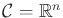, as in Section 7.4.1. This means that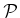includes only polynomials that encode closure constraints, as opposed to allowing constraints that represent rotations. Using the tree representation from Section 4.4.3, this means thatis of dimension, arising from assigning one variable for each revolute joint of the linkage in the absence of any constraints. Let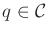denote this vector of configuration variables. The active-passive decomposition partitions the variables of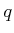to form two vectors,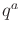, called the active variables and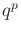, called the passive variables. The values of passive variables are always determined from the active variables by enforcing the closure constraints and using inverse kinematics techniques. If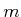is the dimension of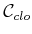, then there are alwaysactive variables and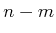passive variables.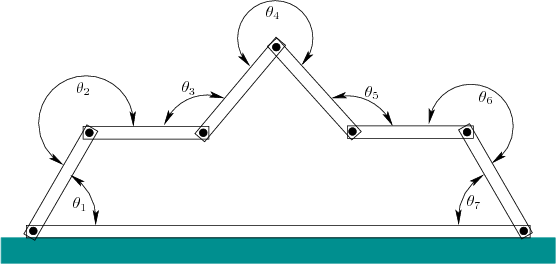Temporarily, suppose that the linkage forms a single loop as shown in Figure 7.23. One possible decomposition into activeand passivevariables is given in Figure 7.24. If constrained to form a loop, the linkage has four degrees of freedom, assuming the bottom link is rigidly attached to the ground. This means that values can be chosen for four active joint anglesand the remaining threecan be derived from solving the inverse kinematics. To determine, there are three equations and three unknowns. Unfortunately, these equations are nonlinear and fairly complicated. Nevertheless, efficient solutions exist for this case, and the 3D generalization . For a 3D loop formed of revolute joints, there are six passive variables. The number,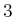, of passive links in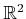and the number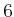for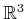arise from the dimensions of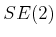and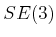, respectively. This is the freedom that is stripped away from the system by enforcing the closure constraints. Methods for efficiently computing inverse kinematics in two and three dimensions are given in . These can also be applied to the RDT stepping method in Section 7.4.1, instead of using continuation.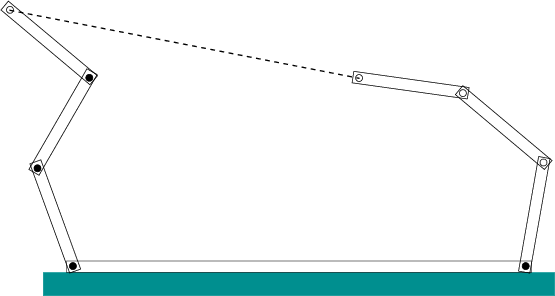If the maximal number of passive variables is used, there is at most a finite number of solutions to the inverse kinematics problem; this implies that there are often several choices for the passive variable values. It could be the case that for some assignments of active variables, there are no solutions to the inverse kinematics. An example is depicted in Figure 7.25. Suppose that we want to generate samples inby selecting random values forand then using inverse kinematics for. What is the probability that a solution to the inverse kinematics exists? For the example shown, it appears that solutions would not exist in most trials.

Steven M LaValle 2020-08-14